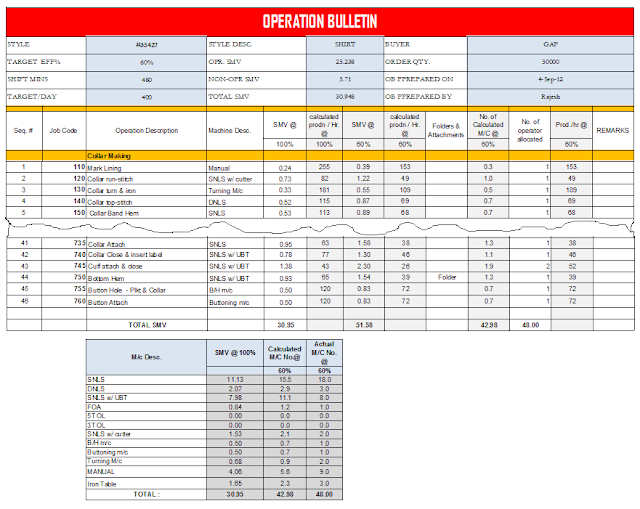# How to Make an Operation Bulletin for a Garment?

An Operation Bulletin is one of the primary IE tools. An OB helps to set a production line with the correct number of machines and manpower. To make it easy for learners I have explained OB making process step by step. Prior to using these steps for making an OB, you need to know how to determine operation SMV, calculation of machine requirement and how to make operation breakdown of a garment.

### Step #1: Prepare an OB format.

You need a computer with a spreadsheet (Excel sheet) to make this format and to do calculations quickly. In the image at the bottom, I have shown one sample operation bulletin. You can copy this format for your use or make one yourself. On the header add details like style#, Buyer, Order Qty. etc.

Add a formula to the cells for auto calculation when you feed SMVs. The formula for each column has been given in the following steps. Cells highlighted with gray colors are set with formula. A basic operation bulletin contains the following information.
• Daily working hours
• Target output per day or per hour
• Total SMV (Sewing SMV and Non-sewing SMV)
• OB prepared by (Name of the Engineer)
• Job code
• Operation description
• Machine description
• SMV @ 100% Efficiency
• SMV @ target efficiency%
• Calculated production per hour
• Name of the Folder and attachment if used
• Calculated no. of machines
• Actual no. of machines
• Estimated production per unit hours
• Any remarks
• Machine summary list

### Step #2: Collect correct Sample

Once you have OB format ready collect your style (reference sample). When you collect a sample from sampling room or merchants make sure that you got approved sample, or you have to do rework later.

### Step #3: Make operation breakdown for the sample

Look into garment construction in details. To have better understanding first break garment into parts (like a collar, front, back etc.). Then list down operations in your notebook according to the section you made. Once you have listed all operations cross-check with garment again and visualize that if all operations are done as per your list you will get an exactly complete garment as per your sample garment. Now enter operations in your format (computer spreadsheet) as per sequence to be performed in line.

### Step #4: Define machine for each operation

Based on seam type used in a style you may need to use different types of sewing machines for different operations and manual workstations. Select one machine and enter into the sheet against each operation. Also, enter name and description of attachments or folder or guides if needed for an operation.

### Step #5: Enter SMVs for each operation

This step is the most critical in term of how you get SMVs, right? You have to find SMVs by conducting time study or existing database. Mention source of SMV (time study or MTM2 database). You can convert SMVs in based on your target line efficiency%. It is optional. In the following example, SMV is shown at 100% efficiency and also converted SMVs into Target efficiency (i.e. 60%). Refer to the formula.

SMV at Target Efficiency = SMV @ 100% efficiency / Target efficiency%

### Step #6: Calculate calculated production per hour

Calculate production per hour at target efficiency%. In the following example, I have shown it at 100% and Target efficiency (60%). Formula used -

Calculated Production/Hour @ target efficiency = 60/Operation SMV @ target Efficiency

### Step #7: Calculate number of machines

To make a garment as per your target you need to install a sufficient number of sewing machines. You will get fraction number of machines (with decimal) in this calculation. In the next column add a number of machines manually round figure for machine numbers. Combine two operations in a single machine where possible, i.e. required machine no. is less or equal to half.

Calculated Machine number = (SMV @ target efficiency * Hourly production target/60)
Or

Calculated Machine number = (SMV @ target efficiency * Daily production target/Total minutes in a shift day)
You can also use pitch time for the calculation of calculated machine number. Formula:

Calculated Machine number =operation SMV / Pitch.

### Step #8: Calculate estimated production per hour

It is calculated according to the machine assigned to each operation. It may be different for few operations than calculated production per hour (step #6) as you set machine numbers to round up value. Formula used -

Estimated Production/Hour = (No. of machine assigned * Hourly target qty.)/Calculated no. of machines

### Step #9: Calculate Machine Summary

At the bottom of the OB add one table for machine summary. This table will help you to quickly find how many actual machines are required for each machine types. In this table add formula to calculate machine summary automatically when you enter the number of machines. See following sample OB sheet.Click on the image to Zoom